Courses

# Ex 2.1 NCERT Solutions - Polynomials Class 10 Notes | EduRev

## Class 10 : Ex 2.1 NCERT Solutions - Polynomials Class 10 Notes | EduRev

The document Ex 2.1 NCERT Solutions - Polynomials Class 10 Notes | EduRev is a part of the Class 10 Course Mathematics (Maths) Class 10.
All you need of Class 10 at this link: Class 10

Exercise 2.1
Ques 1: The graphs of y = p(x) are given in the figure given below, for some polynomials p(x). Find the number of zeroes of p(x), in each case.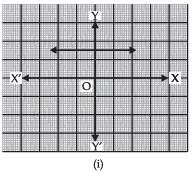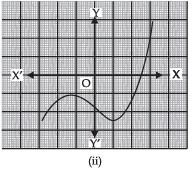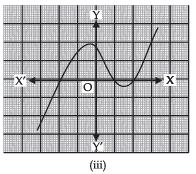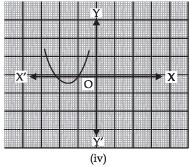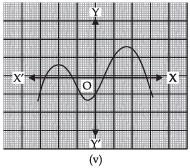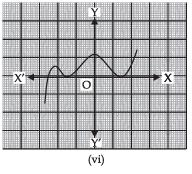Sol. (i) The given graph is parallel to x-axis. It does not intersect the x-axis.
∴ It has no zeroes.
(ii) The given graph intersects the x-axis at one point only.
∴ It has one zero.
(iii) The given graph intersects the x-axis at three points.
∴ It has three zeroes.
(iv) The given graph intersects the x-axis at two points.
∴ It has two zeroes.
(v) The given graph intersects the x-axis at four points.
∴ It has four zeroes.
(vi) The given graph meets the x-axis at three points.
∴ It has three zeroes.

Relationship between Zeroes and Coefficients of a Polynomial
(i) For a quadratic polynomial: If α and β are the zeroes the quadratic polynomial
p (x) = ax2 + bx + c, (a ≠ 0) then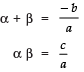i.e.,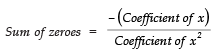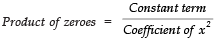(ii) For a cubic polynomial: If α, β, γ are the zeroes of a cubic polynomial ax+ bx2 + cx + d, then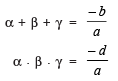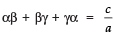i.e.,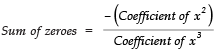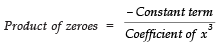Sum of products of the zeroes taken two at a time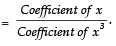Offer running on EduRev: Apply code STAYHOME200 to get INR 200 off on our premium plan EduRev Infinity!

,

,

,

,

,

,

,

,

,

,

,

,

,

,

,

,

,

,

,

,

,

;